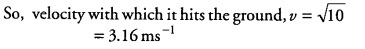# A mass of 10 kg is dropped from a height of 50 cm

A mass of 10 kg is dropped from a height of 50 cm. Find its
(i) potential energy just before dropping.
(ii) kinetic energy just on touching the ground,
(iii) velocity with which it hits the ground.

Given, mass of the object, m = 10 kg , Height, h = 50 cm= 0.5 m
(i) As potential energy is given by PE = mgh
= 10 x 10 X 0.5 = 50 J
(ii) From law of conservation of energy,
total energy of the ball just before dropping = total energy of the ball just on touching the ground => KE + PE of ball just before dropping
= KE of ball just on touching the ground = KE= 50 J
(iii)As , we know KE = 50J
so, 1/2 m {{v}^{2}} = 50
{{v}^{2}} = 50 x 2/10 = 10